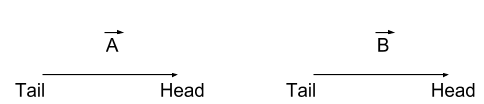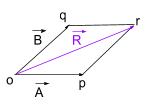Adding two or more vectors is called vector addition. What is a vector? Any quantity which has magnitude along with direction is a vector. Some examples for vector quantities are displacement, velocity, acceleration, force, pressure, etc. Mathematical operations can be performed between two or more vectors. In this article, we will learn about the vector addition of two quantities using the analytical methods.

The process of adding two or more vectors is called vector addition. Depending on the direction of the vector, vector addition is categorized into two types. They are –

• Parallelogram law of vector addition
• Triangular law of vector addition

Consider a vector $$\vec{A}$$ and $$\vec{B}$$The method of vector addition is chosen based on the arrangement of the head and tail of vectors.

• If two vectors are arranged head to tail the triangular law of vector addition is followed.
• If two vectors are arranged head to head or tail to tail then, the parallelogram law of vector addition is followed.

## Parallelogram law of vector addition

If two vectors are arranged head to head or tail to tail then, the parallelogram law of vector addition is carried out.

### Statement

“If two vectors are considered to be the adjacent sides of a parallelogram, then the resultant of two vectors is given by the vector that is a diagonal passing through the point of contact of two vectors.”

### Method

Step-wise vector addition of two vectors using Parallelogram law of vector addition is given below-

Step 1:

Consider two vectors; $$\vec{A}$$ and $$\vec{B}$$Step 2:

Bring the tail of $$\vec{A}$$ to the tail of $$\vec{B}$$. Here the direction of vectors is not changed.Step 3:

Draw a lines parallel to $$\vec{A}$$ and $$\vec{B}$$ with the same magnitude, in a way to complete parallelogram.Step 4:

Join the point o and r by a straight line with an arrow pointing towards the r. This is diagonal to the parallelogram.And this is the resultant vector $$\vec{R}$$ $$\vec{A}+\vec{B}=\vec{R}$$

## Triangular law of vector addition.

If two vectors are arranged head to tail the triangular law of vector addition is carried out.

### Statement

“When two vectors are represented by two sides of a triangle in magnitude and direction were taken in the same order then the third side of that triangle represents in magnitude and direction the resultant of the vectors.”

### Method

Step-wise vector addition of two vectors using Triangular law of vector addition is given below-

Step 1:

Consider two vectors; $$\vec{A}$$ and $$\vec{B}$$Step 2:

Bring the head of $$\vec{A}$$ to the tail of $$\vec{B}$$. Here the direction of vectors is not changed.Step 3:

Join the tail of $$\vec{A}$$ to the head of $$\vec{B}$$ by a straight line with an arrow pointing towards the head of $$\vec{B}$$This new vector is the resultant vector $$\vec{C}$$ $$\vec{A}+\vec{B}=\vec{C}$$

## Why vector addition is important?

In physics, vector quantities like force interact with each other and produce a resultant effect on the objects upon which they are applied. Since the impact of all these forces is taken into consideration when finding the nature of the motion of the system, so, in order to find the resultant of these forces, operations such as addition, subtraction and multiplication are required to be performed on these forces.

## Practice questions on Vector addition

Ans: The process of adding two or more vectors is called vector addition.

Q2: why vector addition is important in physics?

Ans: Physics comprises plenty of vector quantities. When they are acting on a body simultaneously, to find the resultant effect of them on the object vector addition is applied.

Q3: What is a vector?

Ans: A vector is a quantity that has both magnitude and direction.

Q4: Name some vector quantities in physics.

Ans: Some vector quantities in physics are velocity, force, pressure, acceleration.

Q5: Name two types of vector addition.

Ans: Two types of vector addition are- Parallelogram law of vector addition and triangular law of vector addition.

Q6: When do we use triangular law of vector addition?

Ans: When two vectors are arranged in a head-to-tail fashion. The triangular law of vector addition is implemented to calculate the resultant vector.

Q7: When do we use parallelogram law of vector addition?

Ans: When the tail of the two vectors is joined to a common point or head of the two vectors is joined to a common point. The parallelogram law of vector addition is implemented to calculate the resultant vector.

Q8: State parallelogram law of vector addition.

Ans: If two vectors are considered to be the adjacent sides of a parallelogram, then the resultant of two vectors is given by the vector that is a diagonal passing through the point of contact of two vectors.

Q9: State triangular law of vector addition.

Ans: When two vectors are represented by two sides of a triangle in magnitude and direction were taken in the same order then the third side of that triangle represents in magnitude and direction the resultant of the vectors.

Q10: In parallelogram law of vector addition, what represents the resultant vector?

Ans: In parallelogram law of vector addition, diagonal represents the resultant vector.

Hope you have learned about vector addition by parallelogram law of vector addition and triangular law of vector addition along with the statement, step by step method.

Physics Related Topics:

Stay tuned with BYJU’S for more such interesting articles. Also, register to “BYJU’S-The Learning App” for loads of interactive, engaging physics-related videos and an unlimited academic assist.